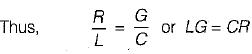Courses

# Transmission Lines

## 10 Questions MCQ Test Topicwise Question Bank for GATE Electrical Engineering | Transmission Lines

Description
This mock test of Transmission Lines for Electrical Engineering (EE) helps you for every Electrical Engineering (EE) entrance exam. This contains 10 Multiple Choice Questions for Electrical Engineering (EE) Transmission Lines (mcq) to study with solutions a complete question bank. The solved questions answers in this Transmission Lines quiz give you a good mix of easy questions and tough questions. Electrical Engineering (EE) students definitely take this Transmission Lines exercise for a better result in the exam. You can find other Transmission Lines extra questions, long questions & short questions for Electrical Engineering (EE) on EduRev as well by searching above.
QUESTION: 1

### For a reciprocal transmission line, the three transmission parameters are given by A = 4, B = 7 and C = 5. The value of D is equal to

Solution:

Since the transmission line is reciprocal, therefore,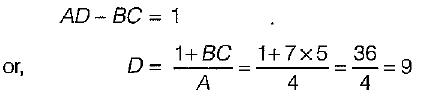QUESTION: 2

Solution:
QUESTION: 3

### In a transmission line terminated by characteristic impedance, Z0

Solution:

When a transmission line is terminated by an impedance Z, then reflection coefficient are: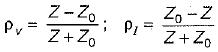Here, Z0 = Characteristic impedance of transmission line.
Since Z = Z0 therefore reflection co-efficient of voltage (pv) and current (pI) both will be zero, i.e there will be no reflection of incident wave.

QUESTION: 4

A symmetrical two-part network have

Solution:
QUESTION: 5

A pure resistance, RL when connected at the load end of a loss-less 100 Ω line produces a VSWR of 2. The RL would be

Solution: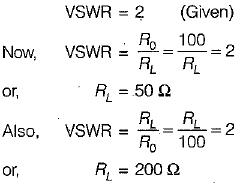QUESTION: 6

The reflection coefficient of a transmission line with a short-circuited load is

Solution:

reflection coefficient of voltage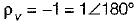and reflection coefficient of current,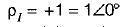Here, only option (b) matches the answer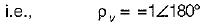QUESTION: 7

'The characteristic impedance Z0 of a transmission line is given by (where R, L, G, C are the unit length parameters)

Solution:

The characteristic impedance (Z0) of a transmission line is given by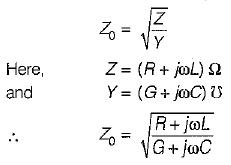QUESTION: 8

The standing wave ratio (VSWR) in terms of reflection coefficient ρ is given by

Solution:
QUESTION: 9

If a transmission line of length less than λ/4  is short circuited, it behaves as

Solution:
QUESTION: 10

A line becomes distortionless

Solution:

A distortionless transmission line is one in which the attenuation constant α is independent of frequency while the phase.constant β is linearly dependent on frequency.